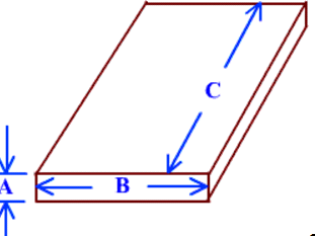Calculating Board Feet of Lumber

Hardwood and Softwood lumber that we use for framing, fencing, cabinetry and furniture is sold by the board foot. This is a measurement that is constant through all thicknesses of lumber, rather than applying to "one-by", "two-by", etc.

In the example piece of lumber below, the measurement "A" would be the thickness in inches , measurement "B" the width in inches, and measurement "C" the length in feet .Thus, the formula for calculating the board feet of lumber in our
example would be;

'A' times "B" times "C" divided by 12 or

For example if the piece if lumber was
3 inches thick (A)
8 inches wide (B)
10 feet long (C), we would have
3 x 8 x 10 divided by 12 = 20 board feet.